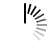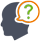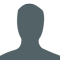Diwali Bonanza | Get 30-Days Free Fun-Filled Learning | Subscribe Now1800-833-6464 Buy Activation Why Tutorix Features Login

#DoubtTushar

In a right-angled triangle with sides $a$ and $b$ and hypotenuse $c$, the altitude drawn on the hypotenuse is $x$. Prove that $ab\ =\ cx$.Solutions :Akhilesh

Given:

In a right-angled triangle with sides $a$ and $b$ and hypotenuse $c$, the altitude drawn on the hypotenuse is $x$.

To do:

We have to prove that $ab\ =\ cx$. ”Š

Solution:

In the given figure, $\vartriangle ABC$ is a right angle triangle having sides $a$ and $b$ and hypotenuse $c$.

Let $BD$ be the altitude drawn on the hypotenuse $AC$.

In $\vartriangle ACB$ and $\vartriangle CDB$,

$\angle B = \angle B$  (Common)

$\angle ACB = \angle CDB = 90^o$

Therefore,

$\vartriangle ACB ∠¼ \vartriangle CDB$ (By AA similarity)

Hence,

$\frac{AB}{BD} = \frac{AC}{BC}$ (Corresponding parts of similar triangles are proportional)

$\frac{a}{x} = \frac{c}{b}$

$ab = cx$

Hence proved.

0

﻿